Quandaries and Queries Name Melanie I am a: Student Level: Middle (8th grade, Algebra 1)   How would you do problems such as these? I have a test tomorrow and this is the only part of the review that I do not understand. Even if you don't answer before the test, your help would be greatly appreciated and helpful. If you can't e-mail the instructions for the graphing part of the question don't worry. I mostly need to know how to solve it.   Solve and Graph the following variable inequalities:   y<2x+4   y>-2x+6   3x+4y<-24   4x-6y>-24                   Thank you so much for your help!                                       Sincerely,                                             Melanie Hi Melanie, First I graphed the equation y = 2x + 4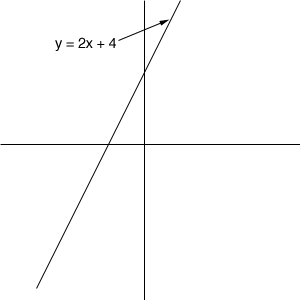Now look at some particular x-value on the x-axis and draw a vertical line through that x-value. The point on the graph directly above (or below) that value (the green dot on the diagram) has its y-value exactly equal to 2x + 4.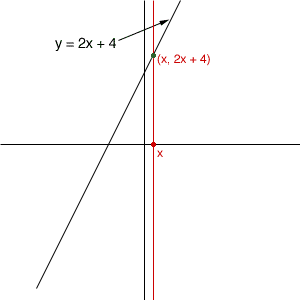The points on that vertical line below the green dot have y-values less than 2x + 4. Thus the points in the plane below the line are the points that satisfy v < 2x + 4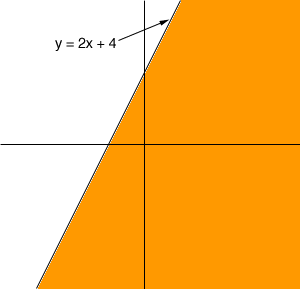Those are the points shaded orange in the diagram above. Once you see what the inequality means you can solve the problem quickly. Suppose we look at y > -2 x + 6. First plot the graph of y = -2 x + 6.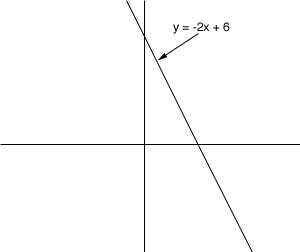You know from above that the solution of y > -2 x + 6 is one side of this line or the other side. But which side? Take some point in the plane which is not on the line. In this problem the easiest point is (0,0). Does (0,0) satisfy the inequality? No it doesn't since 0 < -20 + 6 Thus the solution to y > -2 x + 6 is all points in the plane that are on the side of y > -2 x + 6 that doesn't contain (0,0).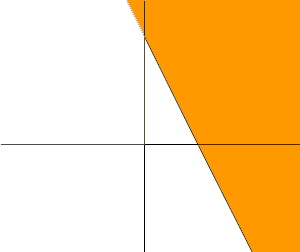Penny Go to Math Central